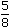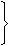Aptitude - Chain Rule

Exercise :: Chain Rule - General Questions

6.

A man completesof a job in 10 days. At this rate, how many more days will it takes him to finish the job?

A. 5
B. 6
C. 7
D.
 7 1 2

Explanation:

 Work done = 5 8

 Balance work =1 - 5= 3 8 8

Let the required number of days be x.

 Then, 5 : 3 = :: 10 : x5 x x = 3 x 10 8 8 8 8x =3 x 10 x 88 5x = 6.

7.

If a quarter kg of potato costs 60 paise, how many paise will 200 gm cost?

 A. 48 paise B. 54 paise C. 56 paise D. 72 paise

Explanation:

Let the required weight be x kg.

Less weight, Less cost (Direct Proportion)250 : 200 :: 60 : x250 x x = (200 x 60)x= (200 x 60) 250x = 48.

8.

In a dairy farm, 40 cows eat 40 bags of husk in 40 days. In how many days one cow will eat one bag of husk?

A. 1
B.
 1 40
C. 40
D. 80

Explanation:

Let the required number of days be x.

Less cows, More days (Indirect Proportion)

Less bags, Less days (Direct Proportion)

 Cows 1 : 40:: 40 : x Bags 40 : 11 x 40 x x = 40 x 1 x 40x = 40.

9.

A wheel that has 6 cogs is meshed with a larger wheel of 14 cogs. When the smaller wheel has made 21 revolutions, then the number of revolutions mad by the larger wheel is:

 A. 4 B. 9 C. 12 D. 49

Explanation:

Let the required number of revolutions made by larger wheel be x.

Then, More cogs, Less revolutions (Indirect Proportion)14 : 6 :: 21 : x14 x x = 6 x 21x = 6 x 21 14x = 9.

10.

If 7 spiders make 7 webs in 7 days, then 1 spider will make 1 web in how many days?

A. 1
B.
 7 2
C. 7
D. 49

Explanation:

Let the required number days be x.

Less spiders, More days (Indirect Proportion)

Less webs, Less days (Direct Proportion)

 Spiders 1 : 7:: 7 : x Webs 7 : 11 x 7 x x = 7 x 1 x 7x = 7.